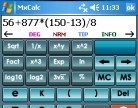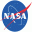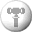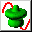# Software SpecificationsView more screenshots
 Editor Rating 0.0
 Version:2.0 Size:3.70 MB License [?]:Shareware Price:\$19.99 USD Last Updated:Jul 27, 2015 Category:Science and Engineering Software Developer:3GR Technologies Operating System:Windows Mobile Additional Requirements:Windows Mobile for PocketPC 2002, 2003, 5.x & 6.x Limitations15-use trial

# EngCalc(Electrical) Review

 " An electrical engineering calculator for PocketPC with unit converter, engineering formulas and evaluator. " This application for Windows Mobile PocketPC has over 100 momentous electrical engineering formulas, comprehensive unit converter, scientific evaluator and Ohms law calculators. EngCalc(Electrical) comes with instant references to recent calculation and solutions to frequently encountered calculations. Features: Over 100 property tables Unit converter with over 1000 units and 10,000 conversions Constant values Scientific evaluator with memory functions, superlative collection of operators and instant reference to recent calculations In-place unit conversion to switch between US and metric units MxCalc with over 2,900 units and new functions (matrices, statistical, financial) Mortgage and loan calculator with amortization tables Periodic chart of Elements Date and time calculations support USA and European date format Over 89 new electrical calculators List of calculators include: Capacitive Energy Capacitor Charge Cylindrical Capacitor Solenoid Magnetic Field Straight Wire Magnetic Field Toroid Magnetic Field Plate Capacitor DC Inductor Voltage Inductive Energy Parallel Capacitance RC Transient RL Transient Series Inductance Series Resistance Coulombs Law Resonant LC Freq Parallel Inductance Parallel Resistance Series Capacitance Ammeter Shunts Capacitance Capacitance Law Cap-Freq-Ind (Inductance) Cap-Freq-Ind (Frequency) Cap-Freq-Ind (Capacitance) Res-Freq-Cap (Capacitance) Res-Freq-Cap (Resistance) Res-Freq-Cap (Frequency) Wheatstone Bridge Power Factor from Power Factor Angle Power Factor from Real Power Resistivity Cap. of a Sphere in Space (Capacitance) Cap. of a Sphere in Space (Radius) Len of Wire AND Freq. of Coil (Len of Wire) Len of Wire AND Freq. of Coil (Freq. of Coil) Capacitance of a Toroid Plate Type or Rolled Capacitor Inductance For Spiral Flat Coils Helical Primary Turns for Coils Potential Divider (Voltage Out) Potential Divider (Resistors) Potential Divider (R1) Potential Divider (R2) Power Calculation-Power Power Calculation-Voltage Power Calculation-Current Power Calculation-Power Dissipated in a Resistor L / C Reactance (Capacitive Reactance) L / C Reactance (Inductive Reactance) RMS Calculations (Pulse Waveform) RMS Calculations (Triangle Waveform) RMS Calculations (Trapezoidal Waveform) Temperature Coefficient Speaker 70 Volt Powered Line Electrical Harmonics Helical Coil Inductance Horsepower Created by a hydraulic motor Impedance & Resonant Capacitor for Coils (Current Cycle US 60 CPS) Impedance & Resonant Capacitor for Coils (Current Cycle EUROPEAN 50 CPS) Jar Capacitance For Coils Motor Estimators (Find Amps) Motor Estimators (Find HP) Motor Estimators (Kva (3 Phase)) Motor calculation (Pump motor) Motor calculation (Lifts, elevators and cranes) Motor calculation (Fan - compressor motors) Operation Amplifier (Inverting Amplifier) Operation Amplifier (Non-inverting Amplifier) Operation Amplifier (Differential Amplifier) Inverting Amplifier Non-inverting Amplifier PCB Trace Width (Internal Layer Results) PCB Trace Width (External Layer Results) Transformer Impedence Reactance of Capacitor Transformer KVA Quick Calculation (Single Phase) Transformer KVA Quick Calculation (Three Phase) T-Pad Resistance H-Pad Resistance Star/Delta Transformation (Star to Delta) Star/Delta Transformation (Delta to Star) CCD Object Size

Tags

Share

 1NASA WorldWind A graphically rich 3D virtual globe for use on desktop computers running Windows. Experience Earth terrain, just as if you were really there. 2Science Fair Ten real-world applications of physics and math, ages 9-16. 33D Grapher Plot and animate 2D and 3D equation and table-based graphs with ease. 4ES-Calc A powerful Calculator and Units Conversion Tool for engineers and scientists. 53DMath Explorer A 3D graph plotting software for math, science and engineering.